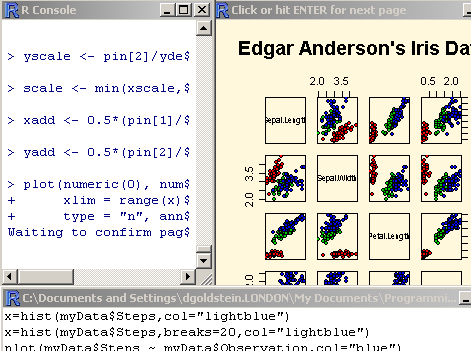Main

## R video tutorial number 1

Filed in Encyclopedia ,RSubscribe to Decision Science News by Email (one email per week, easy unsubscribe)

LEARN R BY JUST WATCHINGFor this week, Decision Science News has created a video tutorial on how to get started using the R Language for Statistical Computing. (The tutorial is best viewed in your browser’s full-screen mode, try pressing F11 in Windows). R is free and open source, and constantly being improved upon by countless contributors worldwide. DSN highly recommends using R.

Hi Res Version at: http://www.dangoldstein.com/flash/Rtutorial1/Rtutorial1.html

Topics covered include:

• The R graphical user interface
• Viewing the graphics demo
• Vectors and basic stats
• Simple plotting

The commands in the tutorial are:
demo(graphics)
x=c(1,2,3,4,5,6,7)
y=c(10,14,20,18,16,15,10)
x+y
z=c(x,y)
sum(y)
mean(y)
sd(y)
plot(x,y)
barplot(y,col=”lightgreen”)

Got that? Now try R video tutorial 2